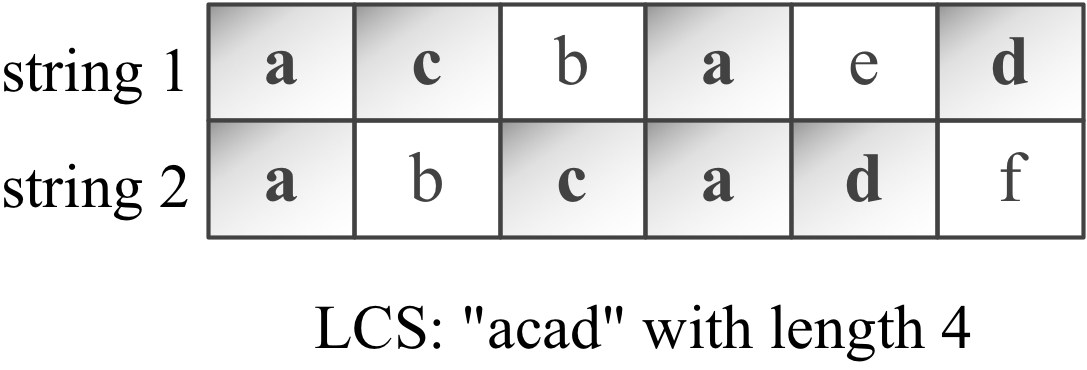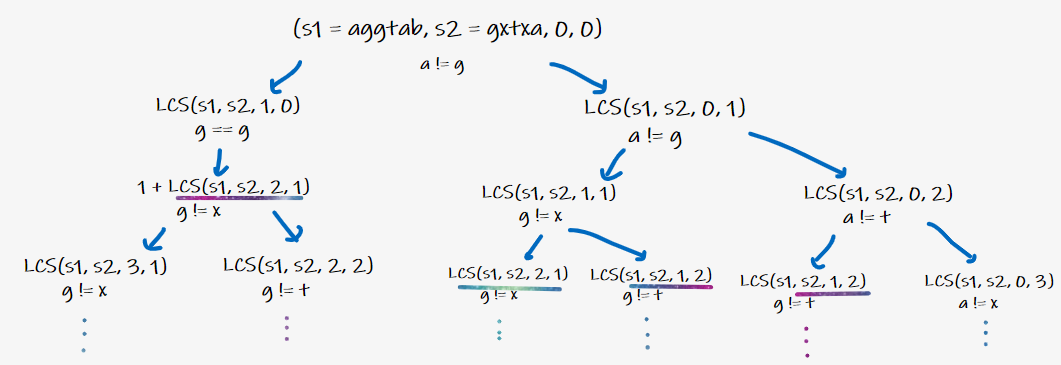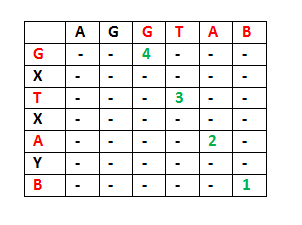# Longest Common Subsequence

## Introduction

Today, let’s learn about a famous and commonly asked Interview Problem of Data Structure, i.e., the Longest Common Subsequence.

It is a famous and common problem that is solved using dynamic programming in its most optimized version.

I know the Dynamic programming problem is a little tricky to understand starting, but if you go step by step in this blog, the problem will be much clear for you to solve other problems based on a similar concept.

Source: Giphy

## Problem Statement

Given two strings, s1 and s2, find the length of their longest common subsequence. If there is no common subsequence, return 0.

A subsequence of a string is a new string generated from the original string with some (or none) characters deleted without changing the relative order of the remaining characters.

So, if a string is “abcde,” some of the possible subsequences of the given string are “cde,” “bc,” etc.

However, “bae” is not a valid subsequence as the relative ordering of characters is not maintained.

Example:

Let the two strings be “acbaed” and “abcadf.”

The longest Common Subsequence of the strings is “acad,” as this subsequence is present in both string1 and string2 and is the longest one.

So, the length of Longest Common Subsequence = size of “acad” = 4.Source: blogspot.com

Let's consider another example: Let the two strings be “acb”  and “dfe”.

The longest Common Subsequence of the strings is “” (empty string) as it has no subsequence, which is present in both string1 and string2.

So, the length of Longest Common Subsequence = length of “” = 0.

Now, let's get started and learn various approaches to solve this problem.

## Approach1 - Using Recursion

The naive (or brute force) solution to this problem could be finding all possible configurations of different subsequences and finding the longest common subsequence possible.

So, we maintain two indexes, i and j, one for each string and increment one by one to find the best possible solution.

Note: If any of the indices reach any of the string’s length, the longest common subsequence would be 0 as the range to find the longest common subsequence.

Implementation

Let’s have a look at its implementation in Java:

``````import java.util.*;
import java.lang.*;
import java.io.*;

class Solution {

// Using recursive approach
public static int LCS(String s1, String s2, int i, int j){

// Base case
if (i == s1.length() || j == s2.length()) return 0;

if (s1.charAt(i) == s2.charAt(j)){
return 1 + LCS(s1, s2, i + 1, j + 1);
}

int option1 = LCS(s1, s2, i + 1, j);
int option2 = LCS(s1, s2, i, j + 1);

return Math.max(option1, option2);
}

public static void main (String[] args){
Scanner s = new Scanner(System.in);

// Take Input
System.out.println("Enter String1");
String s1 = s.next();

System.out.println("Enter String2");
String s2 = s.next();

int lcsans = LCS(s1,s2,0,0);

System.out.println("Longest Common Subsequence "+ lcsans);
}
}``````

Output:

## Time and Space Complexity

Time Complexity: O(2 ^ N), i.e., exponential as we generate and compare all the subsequences of both the strings.

Note: The total number of subsequences of a string is O(2 ^ N).

Space Complexity: O(1) as no extra space is being used.

Where ‘N’ is the length of the shortest of the two strings.

## Approach 2 - Using Dynamic Programming

We could optimize the time complexity of our previous approach by maintaining a “dp array” where - dp[i][j] stores the longest common subsequence value that could be formed via considering string1 till i-th index and string2 till jth index.

Let's look at the recursive tree:Since the recursive approach had a lot of overlapping subproblems, the dp array would also help us avoid that repetitive work.

So, let’s consider the two strings “aggtab” and “gxtxayb.”

“-” here indicates that the value of LCS = 0 after considering the string1 and string2 till ith and jth index, respectively.

Every time a matching character is found, the LCS value increases by one else; it remains as it is.APPROACH 2a: Using Top-Down Dp

Implementation

Let’s have a look at its implementation in Java

``````import java.util.*;
import java.lang.*;
import java.io.*;

class Solution {

// Using Top Down dp approach
public static int LCS(String s1, String s2, int m, int n, int[][] dp){

// Base Case
if (m <= 0 || n <= 0) return 0;

// LookUp
if (dp[m][n] != 0) return dp[m][n];

// Calculating Longest Common Subsequence
if (s1.charAt(m) == s2.charAt(n))
dp[m][n] = LCS(s1, s2, m - 1, n - 1, dp) + 1;
else
dp[m][n] = Math.max(LCS(s1, s2, m - 1, n, dp), LCS(s1, s2, m, n - 1, dp));

return dp[m][n];
}

public static void main (String[] args){
Scanner s = new Scanner(System.in);

// Take Input
System.out.println("Enter String1");
String s1 = s.next();

System.out.println("Enter String2");
String s2 = s.next();

int m = s1.length();
int n = s2.length();

// Forming dp array
int dp[][] = new int[m][n];

int lcsans = LCS(s1, s2, m - 1, n - 1, dp);

System.out.println("Longest Common Subsequence "+ lcsans);
}
}``````

Output

## Time and Space Complexity

Time Complexity: O(N ^ 2) as for each length, we are traversing from 0 to that particular length to calculate our max possible profit for the current length.

Space Complexity: O(N ^ 2) as extra space is used to store the longest common subsequence value after considering both the strings until a particular index.

Where ‘N’ is the length of the shortest of the two strings.

APPROACH 2b: Using Bottom-Up Dp

Implementation

Let’s have a look at its implementation in Java

``````import java.util.*;
import java.lang.*;
import java.io.*;

class Solution {

// Using dp approach
public static int LCS(String s1, String s2){

int m = s1.length();
int n = s2.length();

// Forming dp array
int dp[][] = new int[m + 1][n + 1];

// Calculating Longest Common Subsequence
for (int i=0; i <= m; i++){
for (int j=0; j <= n; j++){
if (i == 0 || j == 0)
dp[i][j] = 0;

/*
LCS length increases by one
when there is a character match
*/
else if (s1.charAt(i - 1) == s2.charAt(j - 1))
dp[i][j] = dp[i - 1][j - 1] + 1;
else
dp[i][j] = Math.max(dp[i-1][j], dp[i][j-1]);
}
}
return dp[m][n];
}

public static void main (String[] args){
Scanner s = new Scanner(System.in);

// Take Input
System.out.println("Enter String1");
String s1 = s.next();

System.out.println("Enter String2");
String s2 = s.next();

int lcsans = LCS(s1,s2);

System.out.println("Longest Common Subsequence "+ lcsans);
}
}``````

Output:

## Time and Space Complexity

Time Complexity: O(N ^ 2) as for each length, we are traversing from 0 to that particular length to calculate our max possible profit for the current length.

Space Complexity: O(N ^ 2) as extra space is used to store the longest common subsequence value after considering both the strings until a particular index.

Where ‘N’ is the length of the shortest of the two strings.

### What is dynamic programming?

Dynamic programming is both a mathematical and computer programming method mainly used to optimize plain recursion.

### What are two ways to apply dynamic programming?

The two ways to apply dp are bottom-up (i.e., iterative way) and top-down (i.e., using DP in recursion, commonly known as memorization).

### What is a subsequence?

A subsequence of a string is a new string generated from the original string with some (or none) characters deleted without changing the relative order of the remaining characters.

### Why are we optimizing using dp?

This is because the recursive approach had a lot of overlapping subproblems. The DP array would also help us avoid that repetitive work.

## Conclusion

In this blog, we learned various approaches to the Longest Common Subsequence.

• Longest Common Subsequence is a standard recursive problem that is optimized via Dynamic Programming.
• A subsequence of a string is a new string generated from the original string with some (or none) characters deleted without changing the relative order of the remaining characters.
• The optimized time complexity of this problem is O(N ^ 2) for each character at an index, and we are calculating and comparing the LCS value to the ith and jth index value of string1 and string2, respectively.

Refer to the most obliging blog on Dynamic programming for your future references→ Roadmap for Dynamic programming.

Check out more blogs on different Dynamic Programming problems like Longest Common Substring and Friends Pairing Problem to read more about these topics in detail. And if you liked this blog, share it with your friends!

I hope that this blog has helped you enhance your knowledge regarding the above Data Structures and Algorithms problem and if you would like to learn more, check out our articles on the CodeStudio. Also, check out our course on C++ and look at the interview experiences and interview bundle for placement preparations.

Happy Coding!!